# Selina Solutions Concise Maths Class 10 Chapter 5 Quadratic Equations Exercise 5(C)

A quadratic equation can be solved by many methods. In this exercise, students will be solving quadratic equations by factorisation method. For further clarifications regarding this method of solving the Selina Solutions for Class 10 Maths can be used by students. Also, the solutions to this exercise are available in the Concise Selina Solutions for Class 10 Maths Chapter 5 Quadratic Equations Exercise 5(C) PDF in the links provided below.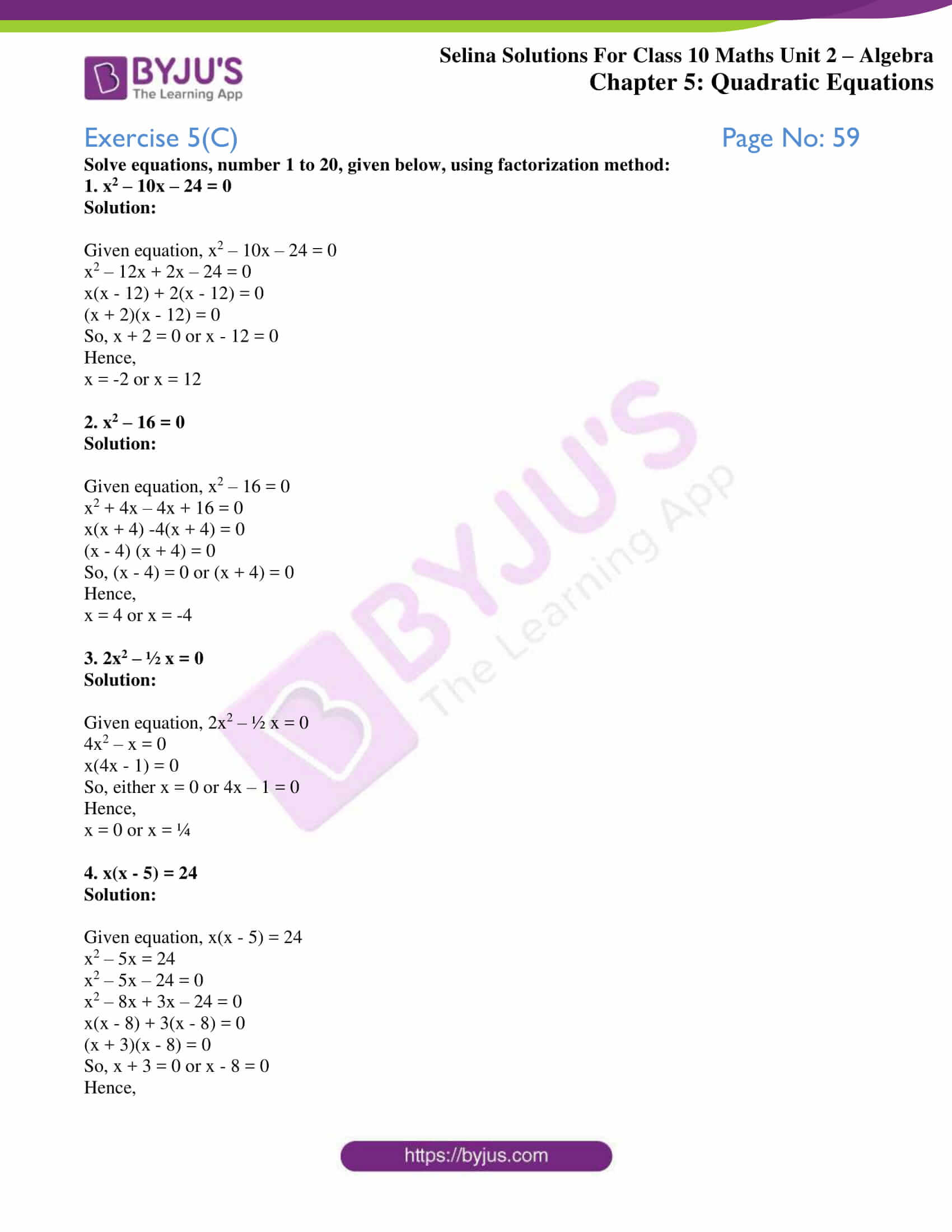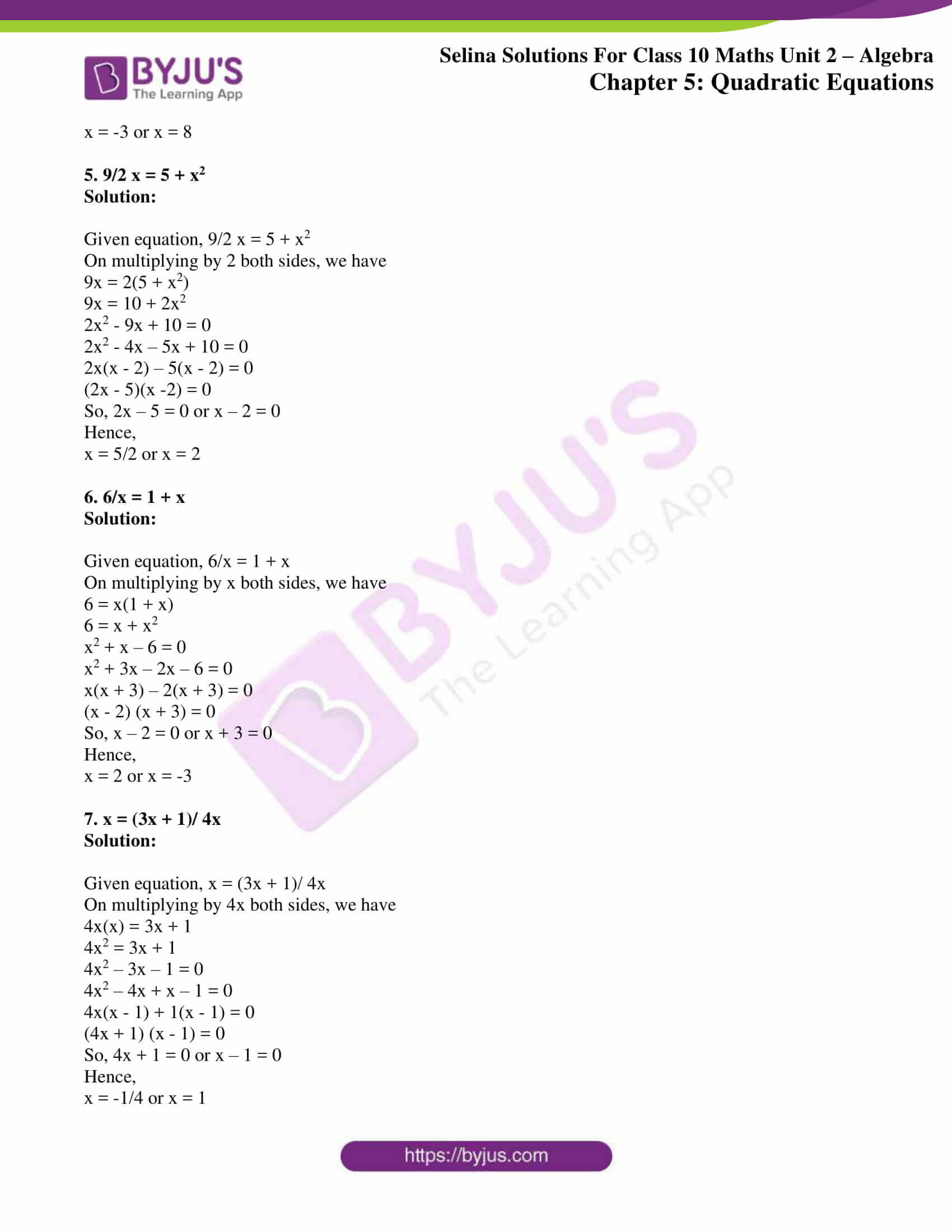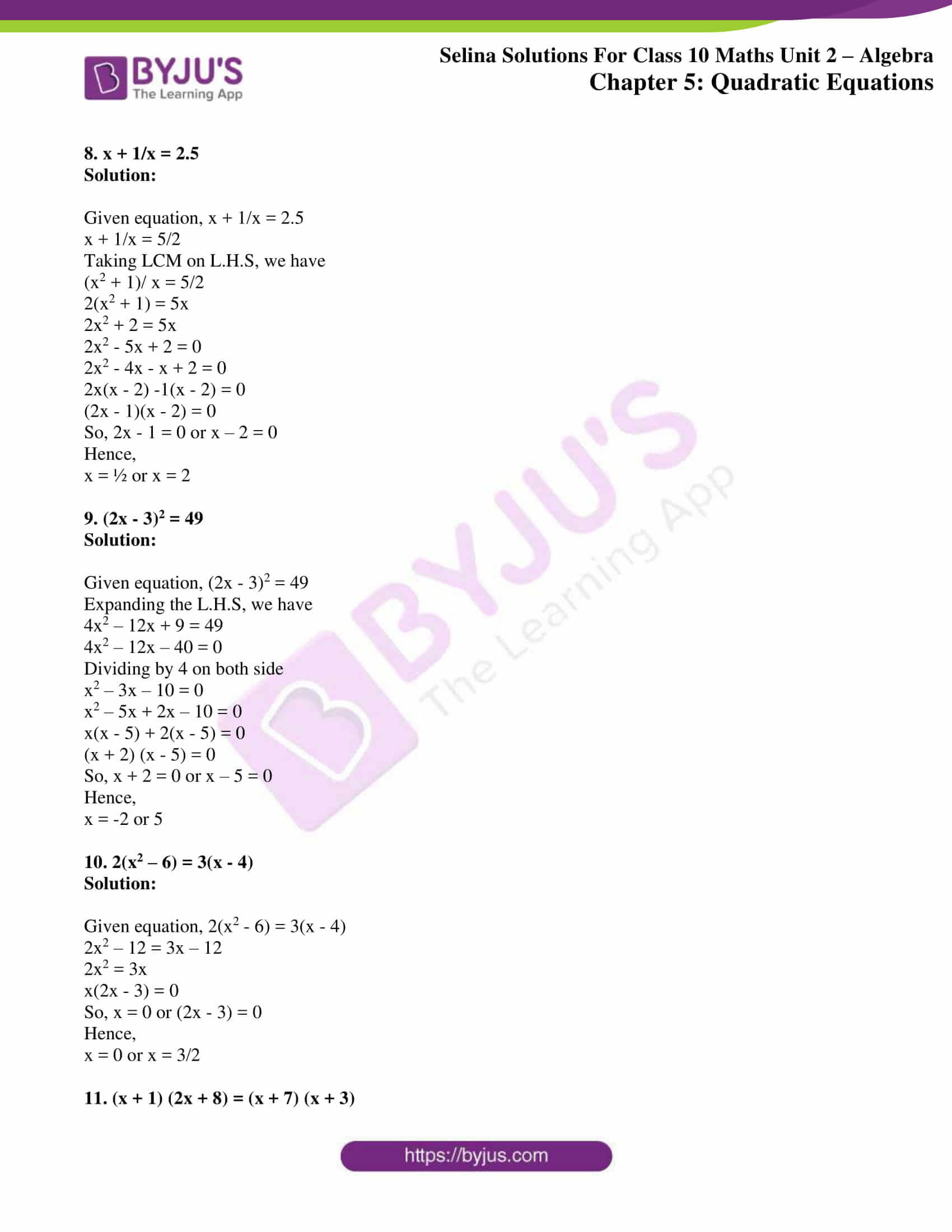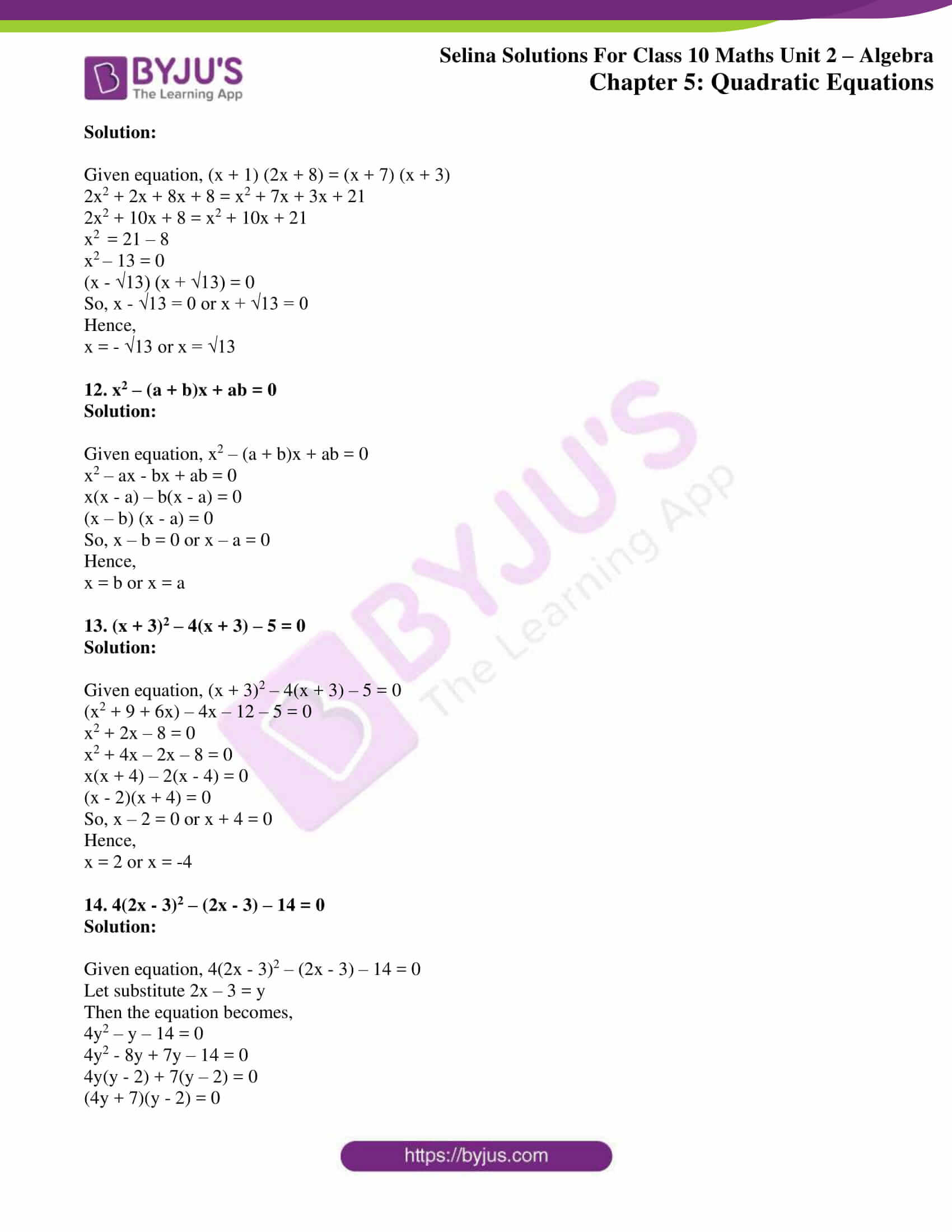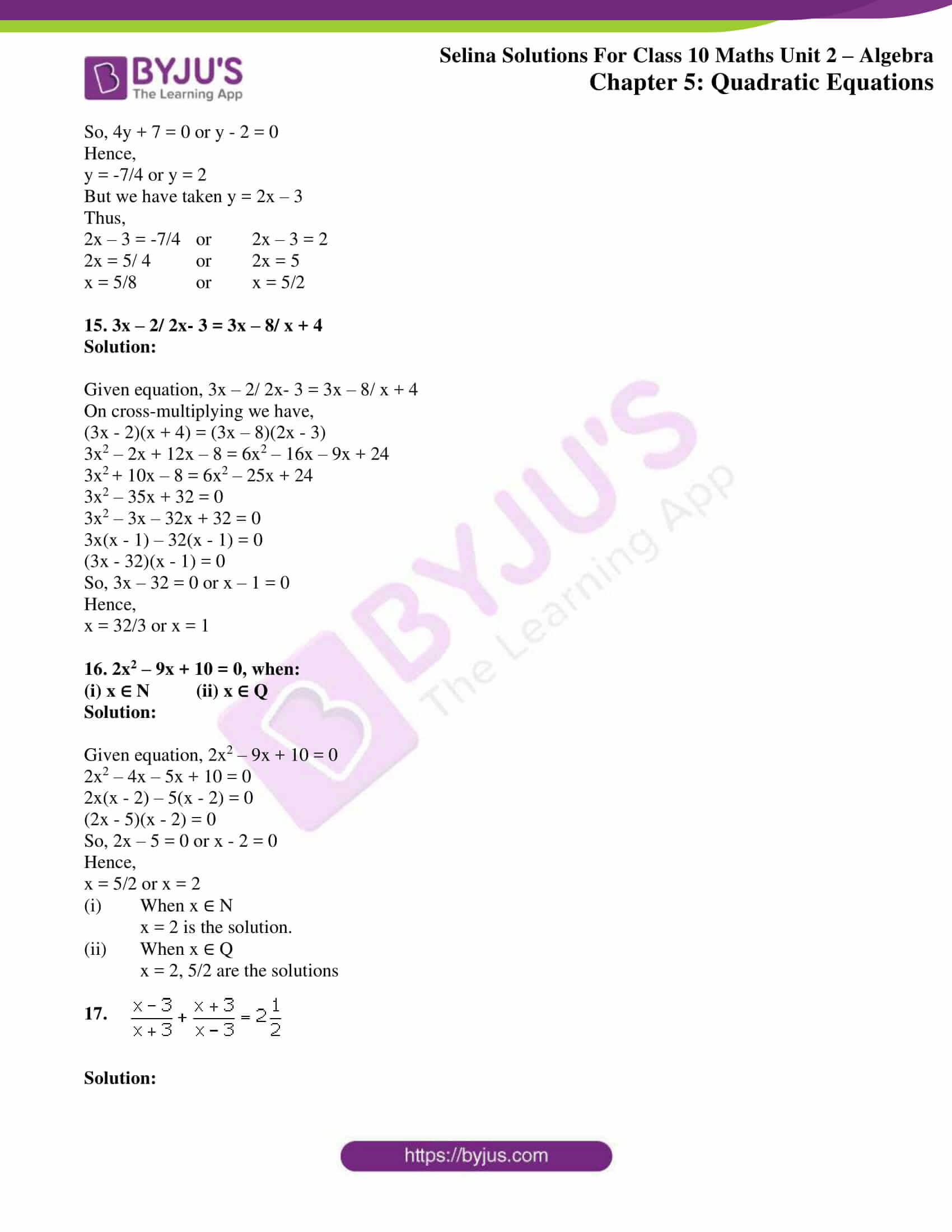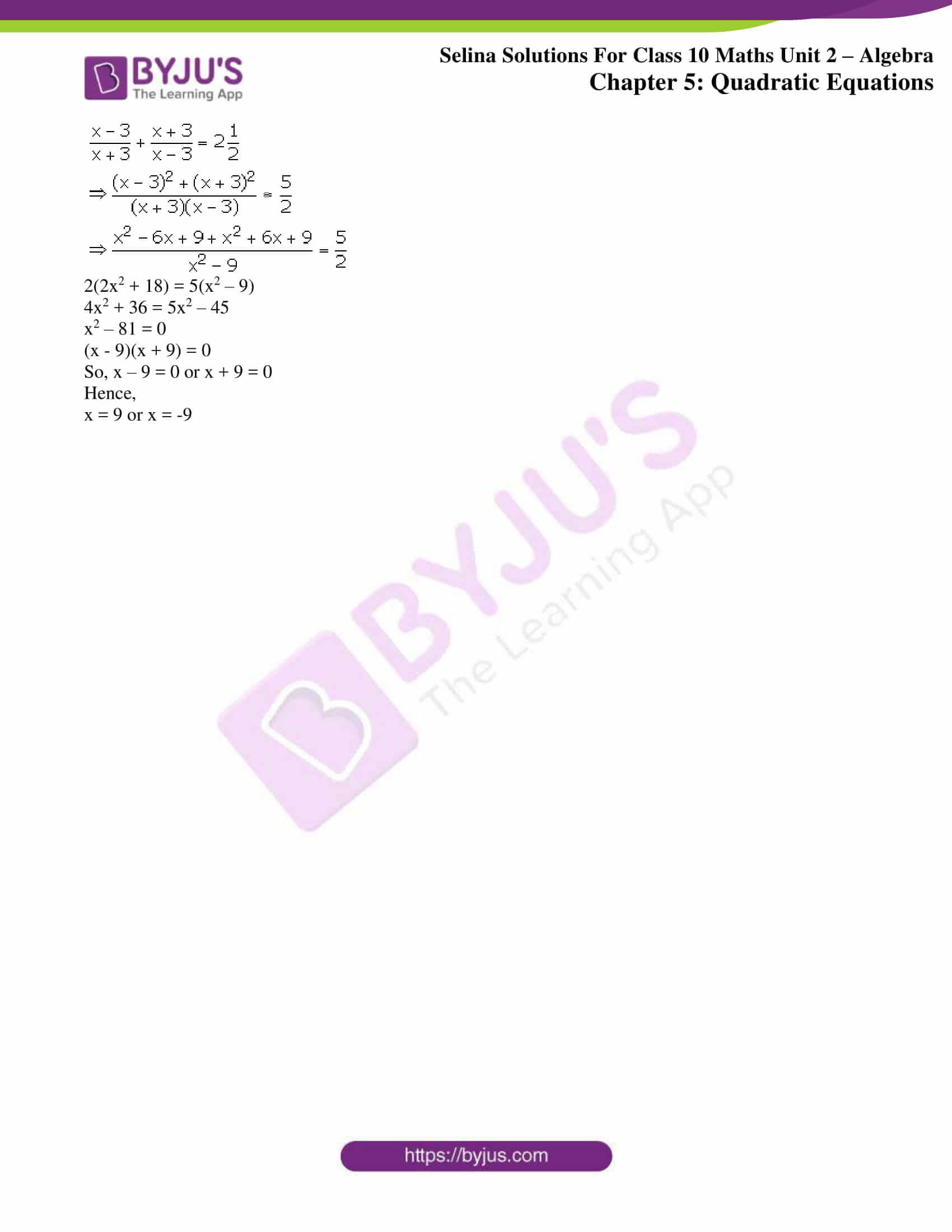### Access other exercises of Selina Solutions Concise Maths Class 10 Chapter 5 Quadratic Equations

Exercise 5(A) Solutions

Exercise 5(B) Solutions

Exercise 5(D) Solutions

Exercise 5(E) Solutions

Exercise 5(F) Solutions

### Access Selina Solutions Concise Maths Class 10 Chapter 5 Quadratic Equations Exercise 5(C)

Solve equations, number 1 to 20, given below, using factorization method:

1. x2 – 10x – 24 = 0

Solution:

Given equation, x2 – 10x – 24 = 0

x2 – 12x + 2x – 24 = 0

x(x – 12) + 2(x – 12) = 0

(x + 2)(x – 12) = 0

So, x + 2 = 0 or x – 12 = 0

Hence,

x = -2 or x = 12

2. x2 – 16 = 0

Solution:

Given equation, x2 – 16 = 0

x2 + 4x – 4x + 16 = 0

x(x + 4) -4(x + 4) = 0

(x – 4) (x + 4) = 0

So, (x – 4) = 0 or (x + 4) = 0

Hence,

x = 4 or x = -4

3. 2x2 – ½ x = 0

Solution:

Given equation, 2x2 – ½ x = 0

4x2 – x = 0

x(4x – 1) = 0

So, either x = 0 or 4x – 1 = 0

Hence,

x = 0 or x = ¼

4. x(x – 5) = 24

Solution:

Given equation, x(x – 5) = 24

x2 – 5x = 24

x2 – 5x – 24 = 0

x2 – 8x + 3x – 24 = 0

x(x – 8) + 3(x – 8) = 0

(x + 3)(x – 8) = 0

So, x + 3 = 0 or x – 8 = 0

Hence,

x = -3 or x = 8

5. 9/2 x = 5 + x2

Solution:

Given equation, 9/2 x = 5 + x2

On multiplying by 2 both sides, we have

9x = 2(5 + x2)

9x = 10 + 2x2

2x2 – 9x + 10 = 0

2x2 – 4x – 5x + 10 = 0

2x(x – 2) – 5(x – 2) = 0

(2x – 5)(x -2) = 0

So, 2x – 5 = 0 or x – 2 = 0

Hence,

x = 5/2 or x = 2

6. 6/x = 1 + x

Solution:

Given equation, 6/x = 1 + x

On multiplying by x both sides, we have

6 = x(1 + x)

6 = x + x2

x2 + x – 6 = 0

x2 + 3x – 2x – 6 = 0

x(x + 3) – 2(x + 3) = 0

(x – 2) (x + 3) = 0

So, x – 2 = 0 or x + 3 = 0

Hence,

x = 2 or x = -3

7. x = (3x + 1)/ 4x

Solution:

Given equation, x = (3x + 1)/ 4x

On multiplying by 4x both sides, we have

4x(x) = 3x + 1

4x2 = 3x + 1

4x2 – 3x – 1 = 0

4x2 – 4x + x – 1 = 0

4x(x – 1) + 1(x – 1) = 0

(4x + 1) (x – 1) = 0

So, 4x + 1 = 0 or x – 1 = 0

Hence,

x = -1/4 or x = 1

8. x + 1/x = 2.5

Solution:

Given equation, x + 1/x = 2.5

x + 1/x = 5/2

Taking LCM on L.H.S, we have

(x2 + 1)/ x = 5/2

2(x2 + 1) = 5x

2x2 + 2 = 5x

2x2 – 5x + 2 = 0

2x2 – 4x – x + 2 = 0

2x(x – 2) -1(x – 2) = 0

(2x – 1)(x – 2) = 0

So, 2x – 1 = 0 or x – 2 = 0

Hence,

x = ½ or x = 2

9. (2x – 3)2 = 49

Solution:

Given equation, (2x – 3)2 = 49

Expanding the L.H.S, we have

4x2 – 12x + 9 = 49

4x2 – 12x – 40 = 0

Dividing by 4 on both side

x2 – 3x – 10 = 0

x2 – 5x + 2x – 10 = 0

x(x – 5) + 2(x – 5) = 0

(x + 2) (x – 5) = 0

So, x + 2 = 0 or x – 5 = 0

Hence,

x = -2 or 5

10. 2(x2 – 6) = 3(x – 4)

Solution:

Given equation, 2(x2 – 6) = 3(x – 4)

2x2 – 12 = 3x – 12

2x2 = 3x

x(2x – 3) = 0

So, x = 0 or (2x – 3) = 0

Hence,

x = 0 or x = 3/2

11. (x + 1) (2x + 8) = (x + 7) (x + 3)

Solution:

Given equation, (x + 1) (2x + 8) = (x + 7) (x + 3)

2x2 + 2x + 8x + 8 = x2 + 7x + 3x + 21

2x2 + 10x + 8 = x2 + 10x + 21

x2 = 21 – 8

x2 – 13 = 0

(x – √13) (x + √13) = 0

So, x – √13 = 0 or x + √13 = 0

Hence,

x = – √13 or x = √13

12. x2 – (a + b)x + ab = 0

Solution:

Given equation, x2 – (a + b)x + ab = 0

x2 – ax – bx + ab = 0

x(x – a) – b(x – a) = 0

(x – b) (x – a) = 0

So, x – b = 0 or x – a = 0

Hence,

x = b or x = a

13. (x + 3)2 – 4(x + 3) – 5 = 0

Solution:

Given equation, (x + 3)2 – 4(x + 3) – 5 = 0

(x2 + 9 + 6x) – 4x – 12 – 5 = 0

x2 + 2x – 8 = 0

x2 + 4x – 2x – 8 = 0

x(x + 4) – 2(x – 4) = 0

(x – 2)(x + 4) = 0

So, x – 2 = 0 or x + 4 = 0

Hence,

x = 2 or x = -4

14. 4(2x – 3)2 – (2x – 3) – 14 = 0

Solution:

Given equation, 4(2x – 3)2 – (2x – 3) – 14 = 0

Let substitute 2x – 3 = y

Then the equation becomes,

4y2 – y – 14 = 0

4y2 – 8y + 7y – 14 = 0

4y(y – 2) + 7(y – 2) = 0

(4y + 7)(y – 2) = 0

So, 4y + 7 = 0 or y – 2 = 0

Hence,

y = -7/4 or y = 2

But we have taken y = 2x – 3

Thus,

2x – 3 = -7/4 or 2x – 3 = 2

2x = 5/ 4 or 2x = 5

x = 5/8 or x = 5/2

15. 3x – 2/ 2x- 3 = 3x – 8/ x + 4

Solution:

Given equation, 3x – 2/ 2x- 3 = 3x – 8/ x + 4

On cross-multiplying we have,

(3x – 2)(x + 4) = (3x – 8)(2x – 3)

3x2 – 2x + 12x – 8 = 6x2 – 16x – 9x + 24

3x2 + 10x – 8 = 6x2 – 25x + 24

3x2 – 35x + 32 = 0

3x2 – 3x – 32x + 32 = 0

3x(x – 1) – 32(x – 1) = 0

(3x – 32)(x – 1) = 0

So, 3x – 32 = 0 or x – 1 = 0

Hence,

x = 32/3 or x = 1

16. 2x2 – 9x + 10 = 0, when:

(i) x ∈ N (ii) x ∈ Q

Solution:

Given equation, 2x2 – 9x + 10 = 0

2x2 – 4x – 5x + 10 = 0

2x(x – 2) – 5(x – 2) = 0

(2x – 5)(x – 2) = 0

So, 2x – 5 = 0 or x – 2 = 0

Hence,

x = 5/2 or x = 2

(i) When x ∈ N

x = 2 is the solution.

(ii) When x ∈ Q

x = 2, 5/2 are the solutions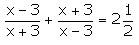17.

Solution: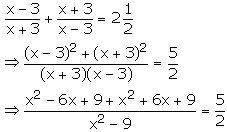2(2x2 + 18) = 5(x2 – 9)

4x2 + 36 = 5x2 – 45

x2 – 81 = 0

(x – 9)(x + 9) = 0

So, x – 9 = 0 or x + 9 = 0

Hence,

x = 9 or x = -9